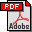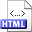A reliable randomized algorithm for the closest-pair problem Authors: Martin Dietzfelbinger, Torben Hagerup, Jyrki Katajainen, and Martti Penttonen Published in: Journal of Algorithms 25,1 (1997), 19-51 Full text:PDF (308 kB) DOI: 10.1006/jagm.1997.0873 Copyright: © Academic Press Abstract: The following two computational problems are studied:Duplicate grouping: Assume that n items are given, each of which is labeled by an integer key from the set {0,…, U − 1}. Store the items in an array of size n such that items with the same key occupy a contiguous segment of the array.Closest pair: Assume that a multiset of n points in the d-dimensional Euclidean space is given, where d ≥ 1 is a fixed integer. Each point is represented as a d-tuple of integers in the range {0,…, U − 1} (or of arbitrary real numbers). Find a closest pair, i. e., a pair of points whose distance is minimal over all such pairs.In 1976 Rabin described a randomized algorithm for the closest-pair problem that takes linear expected time. As a subroutine, he used a hashing procedure whose implementation was left open. Only years later randomized hashing schemes suitable for filling this gap were developed.In this paper, we return to Rabin’s classic algorithm in order to provide a fully detailed description and analysis, thereby also extending and strengthening his result. As a preliminary step, we study randomized algorithms for the duplicate-grouping problem. In the course of solving the duplicate-grouping problem, we describe a new universal class of hash functions of independent interest.It is shown that both of the foregoing problems can be solved by randomized algorithms that use O(n) space and finish in O(n) time with probability tending to 1 as n grows to infinity. The model of computation is a unit-cost RAM capable of generating random numbers and of performing arithmetic operations from the set {+, −, *, div, log2, exp2}, where div denotes integer division and log2 and exp2 are the mappings from IN to IN ∪ {0} with log2(m) = ⌊log2 m⌋ and exp2(m) = 2m, for all m ∈ IN. If the operations log2 and exp2 are not available, the running time of the algorithms increases by an additive term of O(log log U). All numbers manipulated by the algorithms consist of O(log n + log U) bits.The algorithms for both of the problems exceed the time bound O(n) or O(n + log log U) with probability 2−nΩ(1). Variants of the algorithms are also given that use only O(log n + log U) random bits and have probability O(n−α) of exceeding the time bounds, where α ≥ 1 is a constant that can be chosen arbitrarily.The algorithm for the closest-pair problem also works if the coordinates of the points are arbitrary real numbers, provided that the RAM is able to perform arithmetic operations from {+, −, *, div} on real numbers, where a div b now means ⌊a / b⌋. In this case, the running time is O(n) with log2 and exp2 and O(n + log log(δmax / δmin)) without them, where δmax is the maximum and δmin is the minimum distance between any two distinct input points. Related:HTML (Technical report) BibLATEX: ```@article{DHKP1997J, author = {Martin Dietzfelbinger and Torben Hagerup and Jyrki Katajainen and Martti Penttonen}, title = {A reliable randomized algorithm for the closest-pair problem}, journaltitle = {Journal of Algorithms}, volume = {25}, number = {1}, year = {1997}, pages = {19--51}, } ```